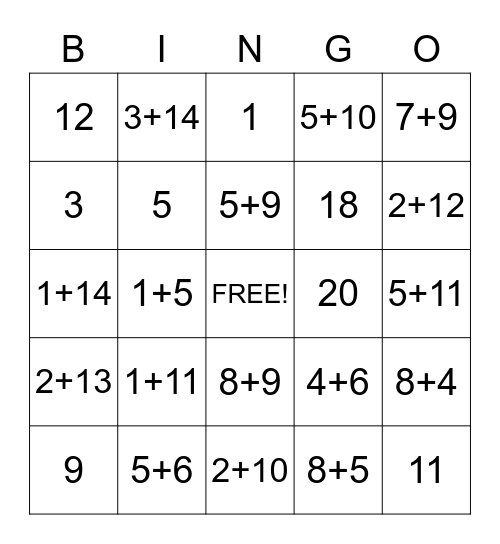This bingo card has a free space and 84 words: 6, 5, 1, 10, 13, 17, 4, 8, 2, 14, 19, 16, 5, 20, 15, 12, 9, 3, 19, 12, 7, 18, 11, 21, 1+5, 1+6, 1+7, 1+8, 1+9, 1+10, 1+11, 1+12, 1+13, 1+14, 1+15, 2+9, 2+10, 2+11, 2+12, 2+13, 2+14, 2+15, 3+10, 3+11, 3+12, 3+13, 3+14, 3+15, 4+2, 4+5, 4+4, 4+6, 5+10, 5+5, 5+8, 5+7, 5+6, 5+9, 5+10, 5+11, 6+10, 6+6, 6+4, 6+14, 7+8, 7+10, 7+4, 7+3, 7+9, 7+2, 8+4, 8+10, 8+11, 8+6, 8+9, 8+5, 8+15, 9+15, 9+14, 9+3, 9+10, 9+11, 9+8 and 9+9.

⚠ This card has duplicate items: 5 (2), 19 (2), 12 (2), 5+10 (2)

## Play Online